Learn Polynomials - Start to Finish - FREE. Check - Polynomials Class 10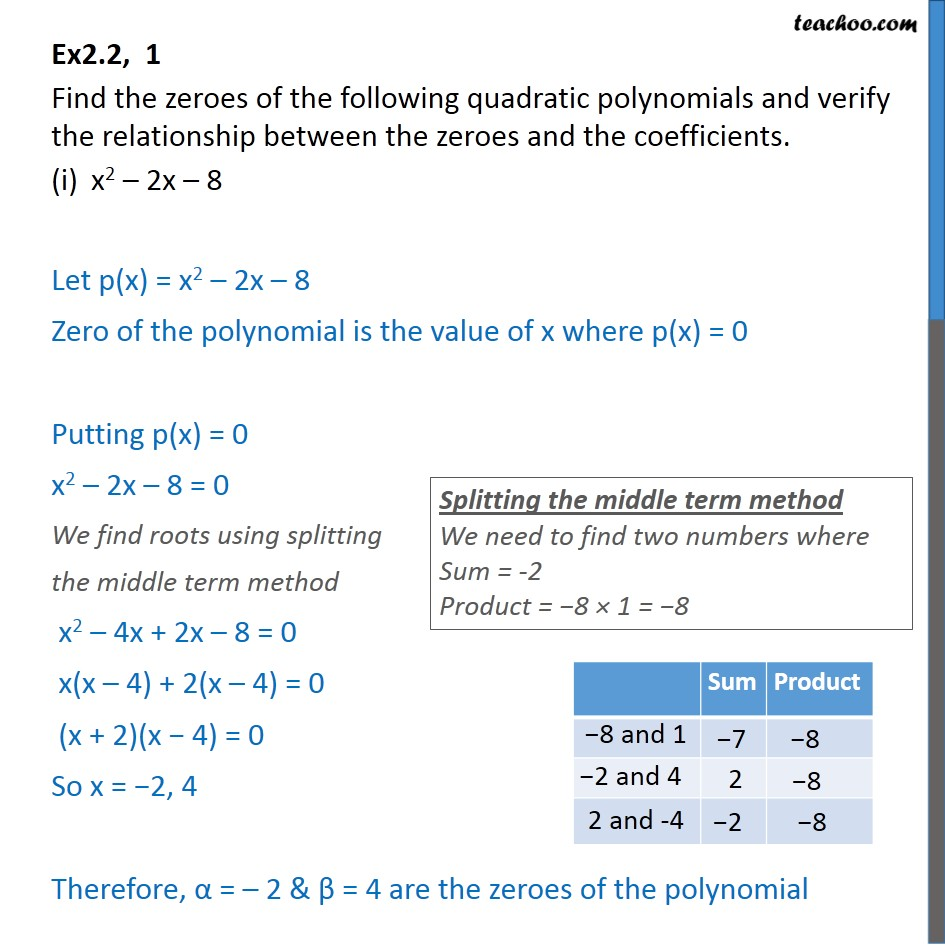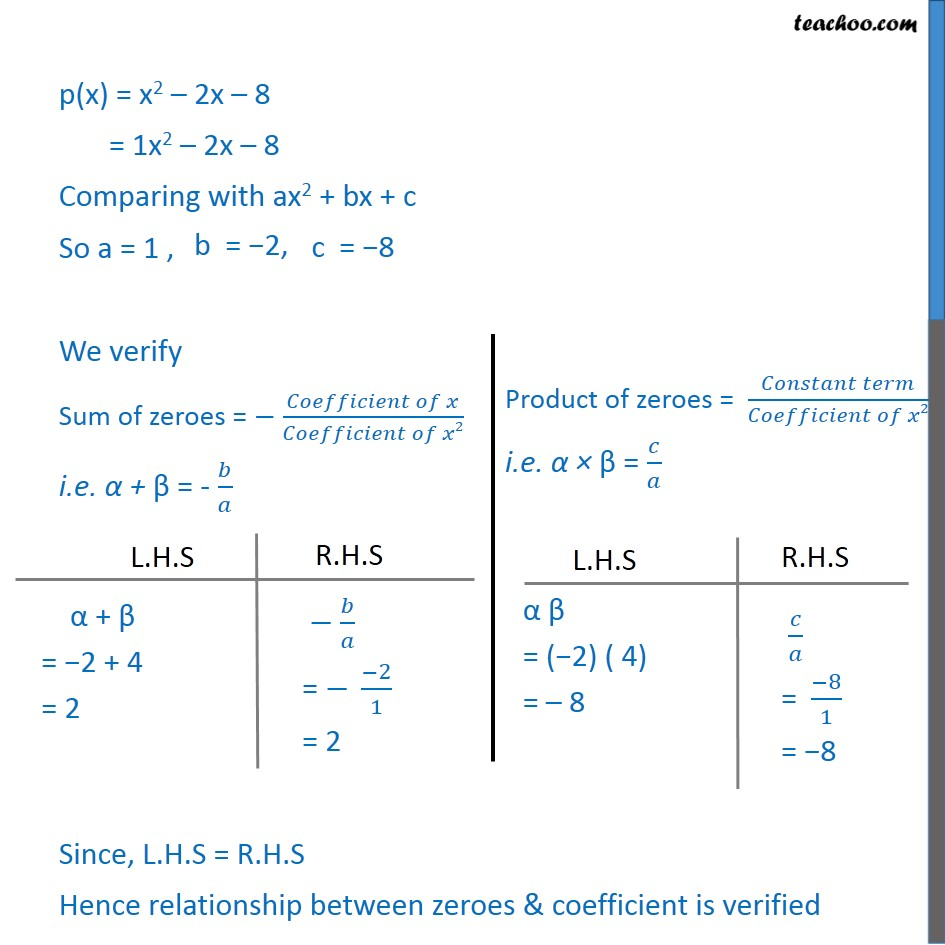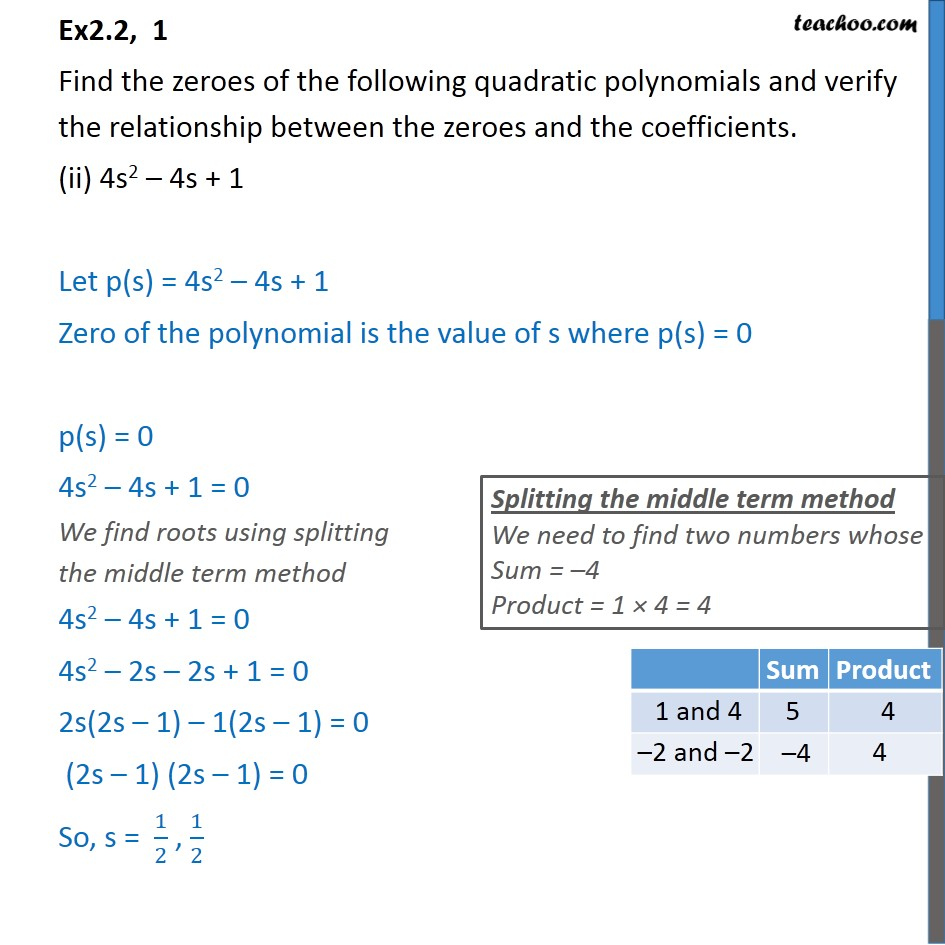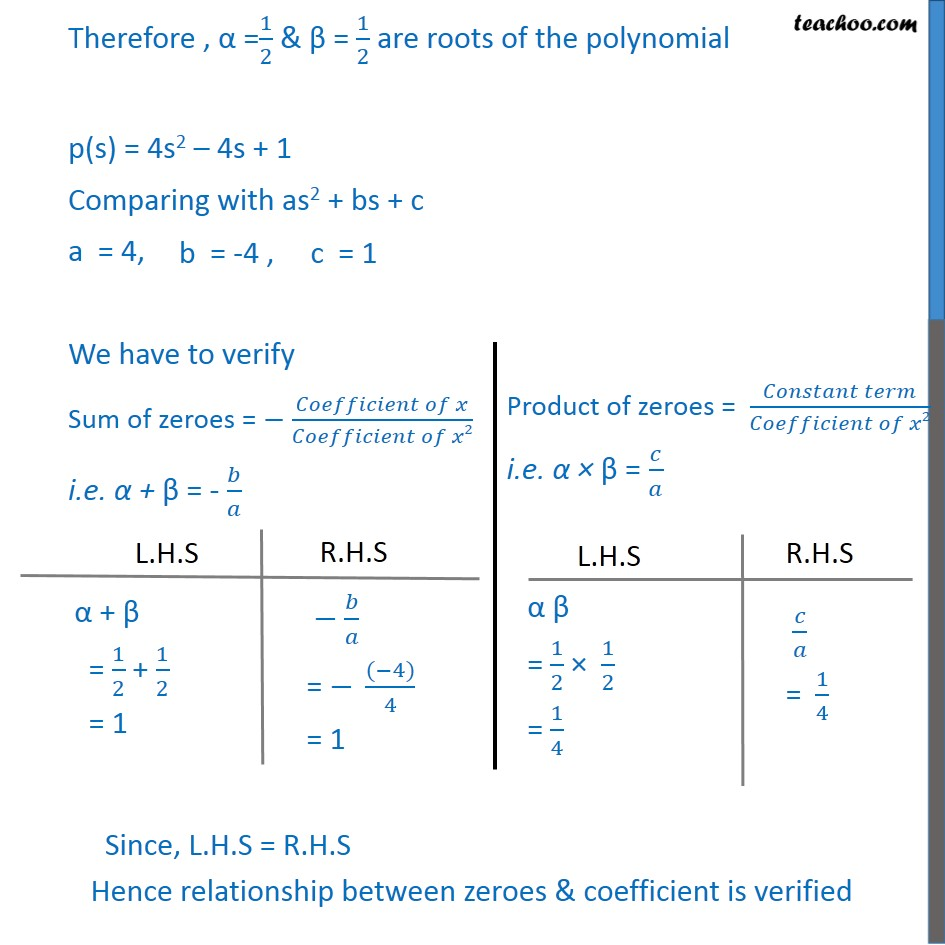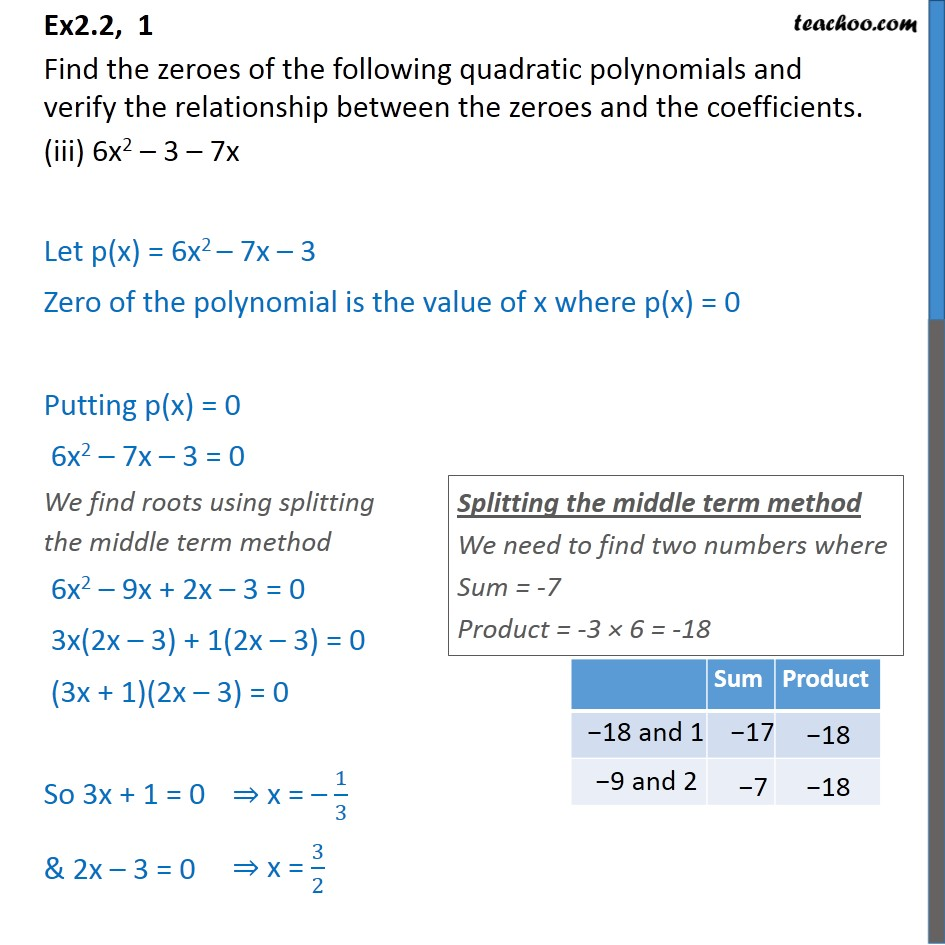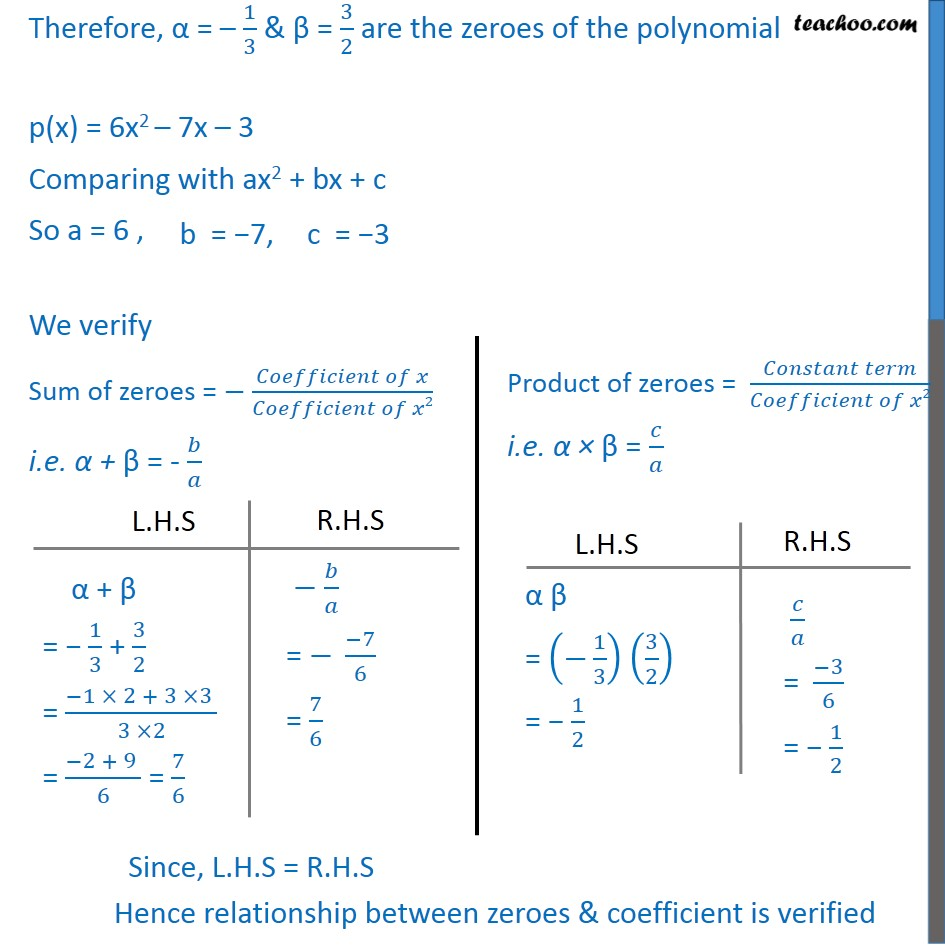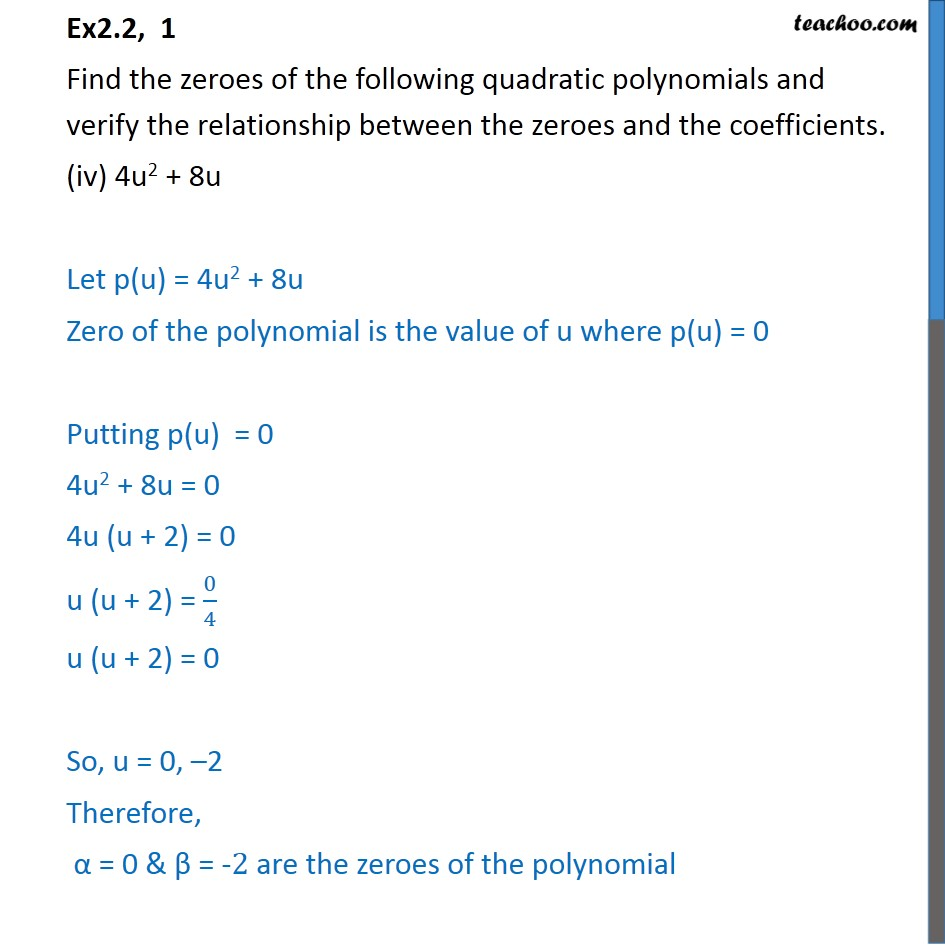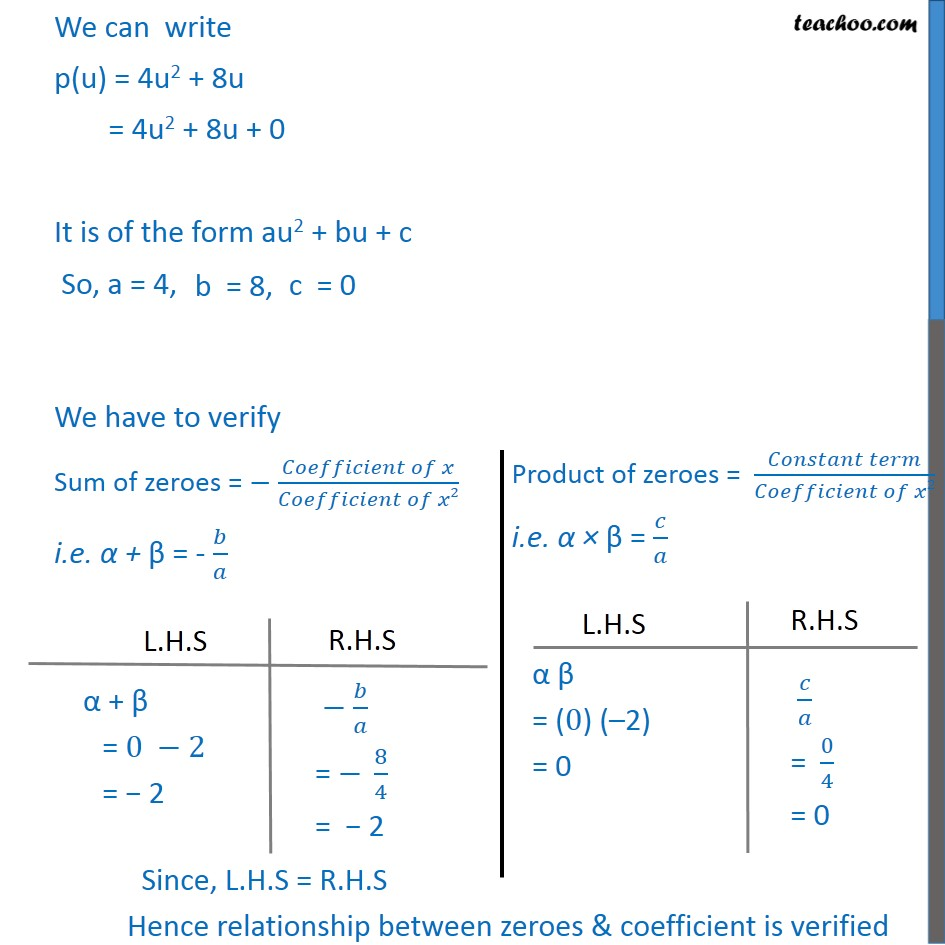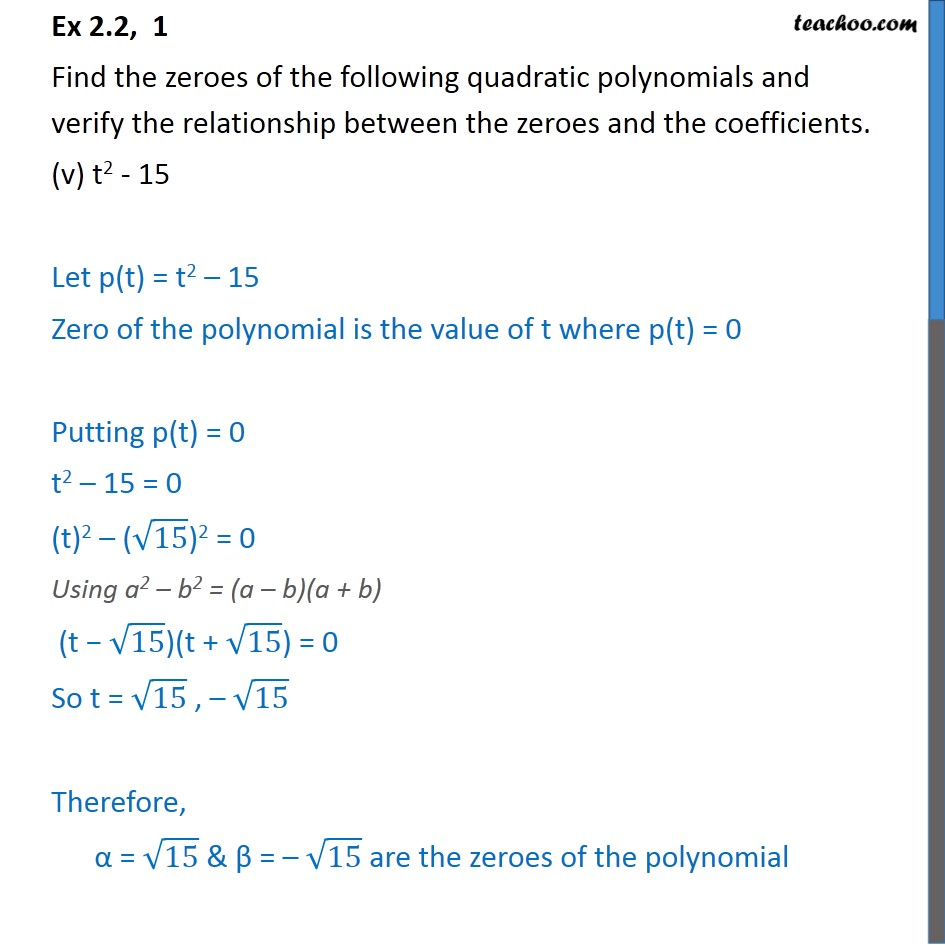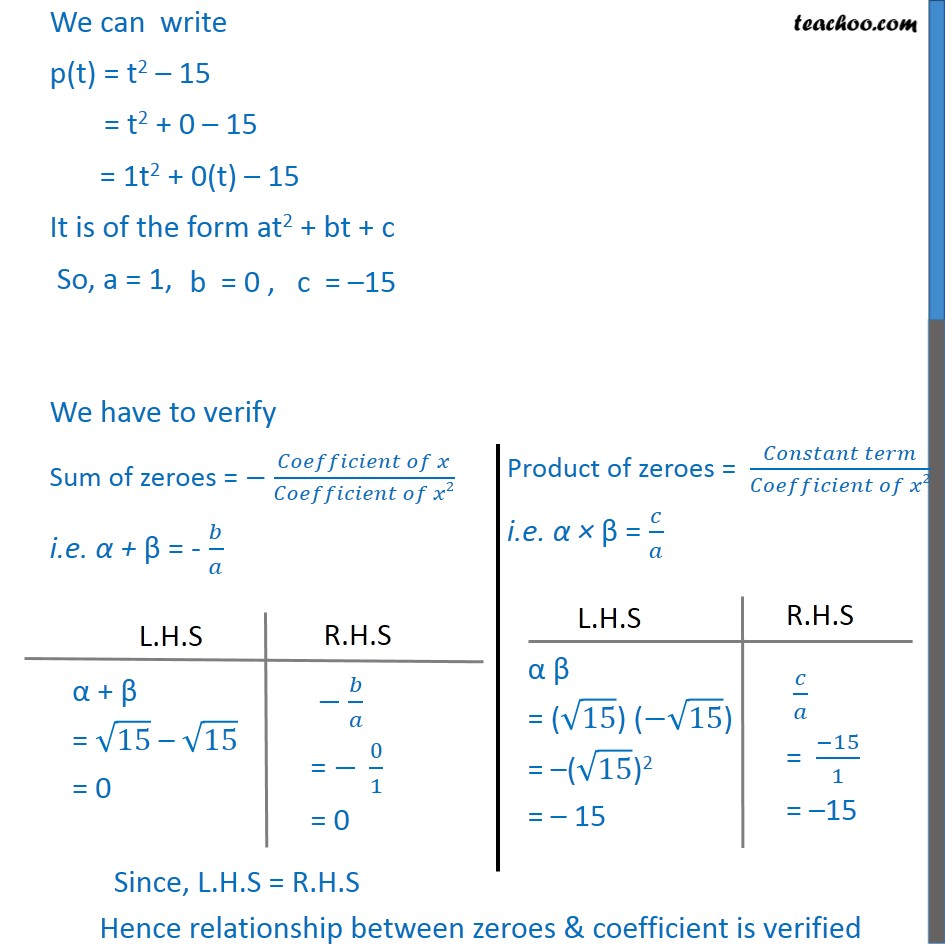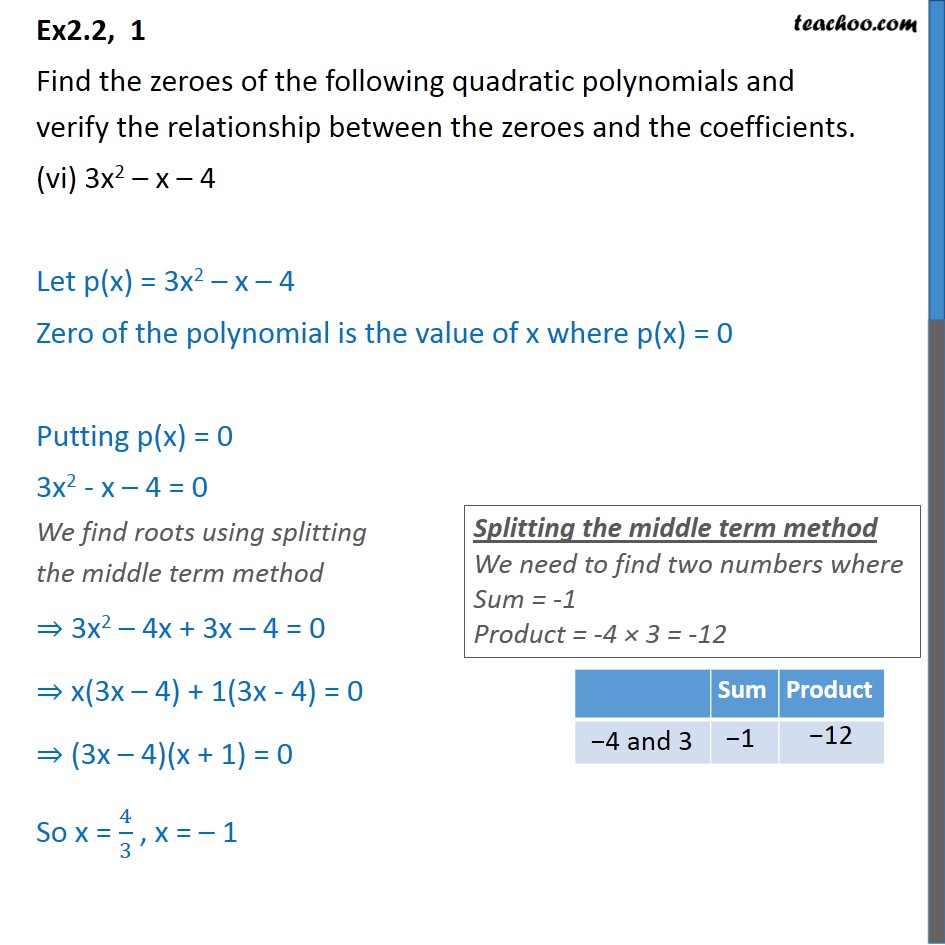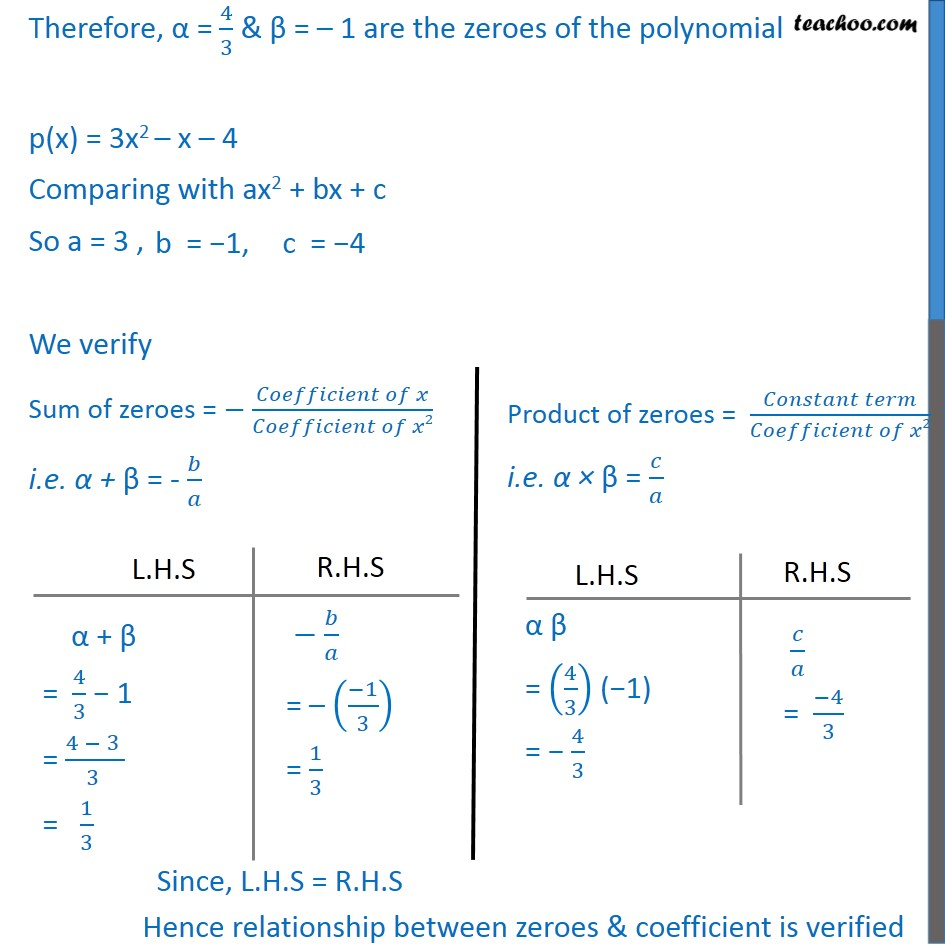1. Chapter 2 Class 10 Polynomials
2. Serial order wise
3. Ex 2.2

Transcript

Ex2.2, 1 Find the zeroes of the following quadratic polynomials and verify the relationship between the zeroes and the coefficients. x2 – 2x – 8 Let p(x) = x2 – 2x – 8 Zero of the polynomial is the value of x where p(x) = 0 Putting p(x) = 0 x2 – 2x – 8 = 0 We find roots using splitting the middle term method x2 – 4x + 2x – 8 = 0 x(x – 4) + 2(x – 4) = 0 (x + 2)(x − 4) = 0 So x = −2, 4 Therefore, α = – 2 & β = 4 are the zeroes of the polynomial p(x) = x2 – 2x – 8 = 1x2 – 2x – 8 Comparing with ax2 + bx + c So a = 1 , We verify Sum of zeroes = − (𝐶𝑜𝑒𝑓𝑓𝑖𝑐𝑖𝑒𝑛𝑡 𝑜𝑓 𝑥)/(𝐶𝑜𝑒𝑓𝑓𝑖𝑐𝑖𝑒𝑛𝑡 𝑜𝑓 𝑥2) i.e. α + β = - 𝑏/𝑎 Since, L.H.S = R.H.S Hence relationship between zeroes & coefficient is verified Ex2.2, 1 Find the zeroes of the following quadratic polynomials and verify the relationship between the zeroes and the coefficients. (ii) 4s2 – 4s + 1 Let p(s) = 4s2 – 4s + 1 Zero of the polynomial is the value of s where p(s) = 0 p(s) = 0 4s2 – 4s + 1 = 0 We find roots using splitting the middle term method 4s2 – 4s + 1 = 0 4s2 – 2s – 2s + 1 = 0 2s(2s – 1) – 1(2s – 1) = 0 (2s – 1) (2s – 1) = 0 So, s = 1/2 , 1/2 Therefore , α =1/2 & β = 1/2 are roots of the polynomial p(s) = 4s2 – 4s + 1 Comparing with as2 + bs + c a = 4, We have to verify Sum of zeroes = − (𝐶𝑜𝑒𝑓𝑓𝑖𝑐𝑖𝑒𝑛𝑡 𝑜𝑓 𝑥)/(𝐶𝑜𝑒𝑓𝑓𝑖𝑐𝑖𝑒𝑛𝑡 𝑜𝑓 𝑥2) i.e. α + β = - 𝑏/𝑎 Ex2.2, 1 Find the zeroes of the following quadratic polynomials and verify the relationship between the zeroes and the coefficients. (iii) 6x2 – 3 – 7x Let p(x) = 6x2 – 7x – 3 Zero of the polynomial is the value of x where p(x) = 0 Putting p(x) = 0 6x2 – 7x – 3 = 0 We find roots using splitting the middle term method 6x2 – 9x + 2x – 3 = 0 3x(2x – 3) + 1(2x – 3) = 0 (3x + 1)(2x – 3) = 0 So 3x + 1 = 0 & 2x – 3 = 0 Therefore, α = – 1/3 & β = 3/2 are the zeroes of the polynomial p(x) = 6x2 – 7x – 3 Comparing with ax2 + bx + c So a = 6 , We verify Sum of zeroes = − (𝐶𝑜𝑒𝑓𝑓𝑖𝑐𝑖𝑒𝑛𝑡 𝑜𝑓 𝑥)/(𝐶𝑜𝑒𝑓𝑓𝑖𝑐𝑖𝑒𝑛𝑡 𝑜𝑓 𝑥2) i.e. α + β = - 𝑏/𝑎 Ex2.2, 1 Find the zeroes of the following quadratic polynomials and verify the relationship between the zeroes and the coefficients. (iv) 4u2 + 8u Let p(u) = 4u2 + 8u Zero of the polynomial is the value of u where p(u) = 0 Putting p(u) = 0 4u2 + 8u = 0 4u (u + 2) = 0 u (u + 2) = 0/4 u (u + 2) = 0 So, u = 0, –2 Therefore, α = 0 & β = -2 are the zeroes of the polynomial We can write p(u) = 4u2 + 8u = 4u2 + 8u + 0 It is of the form au2 + bu + c So, a = 4, We have to verify Sum of zeroes = − (𝐶𝑜𝑒𝑓𝑓𝑖𝑐𝑖𝑒𝑛𝑡 𝑜𝑓 𝑥)/(𝐶𝑜𝑒𝑓𝑓𝑖𝑐𝑖𝑒𝑛𝑡 𝑜𝑓 𝑥2) i.e. α + β = - 𝑏/𝑎 Ex 2.2, 1 Find the zeroes of the following quadratic polynomials and verify the relationship between the zeroes and the coefficients. (v) t2 - 15 Let p(t) = t2 – 15 Zero of the polynomial is the value of t where p(t) = 0 Putting p(t) = 0 t2 – 15 = 0 (t)2 – (√15)2 = 0 Using a2 – b2 = (a – b)(a + b) (t − √15)(t + √15) = 0 So t = √15 , – √15 Therefore, α = √15 & β = – √15 are the zeroes of the polynomial We can write p(t) = t2 – 15 = t2 + 0 – 15 = 1t2 + 0(t) – 15 It is of the form at2 + bt + c So, a = 1, We have to verify Sum of zeroes = − (𝐶𝑜𝑒𝑓𝑓𝑖𝑐𝑖𝑒𝑛𝑡 𝑜𝑓 𝑥)/(𝐶𝑜𝑒𝑓𝑓𝑖𝑐𝑖𝑒𝑛𝑡 𝑜𝑓 𝑥2) i.e. α + β = - 𝑏/𝑎 Ex2.2, 1 Find the zeroes of the following quadratic polynomials and verify the relationship between the zeroes and the coefficients. (vi) 3x2 – x – 4 Let p(x) = 3x2 – x – 4 Zero of the polynomial is the value of x where p(x) = 0 Putting p(x) = 0 3x2 - x – 4 = 0 We find roots using splitting the middle term method ⇒ 3x2 – 4x + 3x – 4 = 0 ⇒ x(3x – 4) + 1(3x - 4) = 0 ⇒ (3x – 4)(x + 1) = 0 So x = 4/3 , x = – 1 Therefore, α = 4/3 & β = – 1 are the zeroes of the polynomial p(x) = 3x2 – x – 4 Comparing with ax2 + bx + c So a = 3 , We verify Sum of zeroes = − (𝐶𝑜𝑒𝑓𝑓𝑖𝑐𝑖𝑒𝑛𝑡 𝑜𝑓 𝑥)/(𝐶𝑜𝑒𝑓𝑓𝑖𝑐𝑖𝑒𝑛𝑡 𝑜𝑓 𝑥2) i.e. α + β = - 𝑏/𝑎

Ex 2.2

Chapter 2 Class 10 Polynomials
Serial order wise